# HC Verma solutions for Class 11, Class 12 Concepts of Physics Vol. 2 chapter 8 - Gauss’s Law [Latest edition]

#### Chapters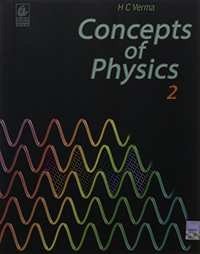## Chapter 8: Gauss’s Law

OthersMCQ
[Page 139]

### HC Verma solutions for Class 11, Class 12 Concepts of Physics Vol. 2 Chapter 8 Gauss’s Law [Page 139]

Q 1 | Page 139

A small plane area is rotated in an electric field. In which orientation of the area, is the flux of the electric field through the area maximum? In which orientation is it zero?

Q 2 | Page 139

A circular ring of radius r made of a non-conducting material is placed with its axis parallel to a uniform electric field. The ring is rotated about a diameter through 180°. Does the flux of the electric field change? If yes, does it decrease or increase?

Q 3 | Page 139

A charge Q is uniformly distributed on a spherical shell. What is the field at the centre of the shell? If a point charge is brought close to the shell, will the field at the centre change? Does your answer depend on whether the shell is conducting or non-conducting?

Q 4 | Page 139

A spherical shell made of plastic, contains a charge Q distributed uniformly over its surface. What is the electric field inside the shell? If the shell is hammered to deshape it, without altering the charge, will the field inside be changed? What happens if the shell is made of a metal?

Q 5 | Page 139

A point charge q is placed in a cavity in a metal block. If a charge Q is brought outside the metal, will the charge q feel an electric fore?

Q 6 | Page 139

A rubber balloon is given a charge Q distributed uniformly over its surface. Is the field inside the balloon zero everywhere if the balloon does not have a spherical surface?

Q 7 | Page 139

It is said that any charge given to a conductor comes to its surface. Should all the protons come to the surface? Should all the electrons come to the surface? Should all the free electrons come to the surface?

MCQ [Pages 139 - 140]

### HC Verma solutions for Class 11, Class 12 Concepts of Physics Vol. 2 Chapter 8 Gauss’s Law MCQ [Pages 139 - 140]

MCQ | Q 1 | Page 139

A charge Q is uniformly distributed over a large plastic plate. The electric field at a point P close to the centre of the plate is 10 V m−1. If the plastic plate is replaced by a copper plate of the same geometrical dimensions and carrying the same charge Q, the electric field at the point P will become

• zero

• 5 V m−1

• 10 V m−1

•  20 V m−1

MCQ | Q 2 | Page 139

A metallic particle with no net charge is placed near a finite metal plate carrying a positive charge. The electric force on the particle will be

• towards the plate

•  away from the plate

•  parallel to the plate

• zero

MCQ | Q 3 | Page 139

A thin, metallic spherical shell contains a charge Q on it. A point charge q is placed at the centre of the shell and another charge q1 is placed outside it as shown in the  following figure . All the three charges are positive. The force on the charge at the centre is ____________.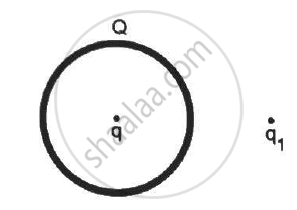•  toward left

•  towards right

• upward

•  zero

MCQ | Q 4 | Page 140

A thin, metallic spherical shell contains a charge Q on it. A point charge q is placed at the centre of the shell and another charge q1 is placed outside it as shown in the following  figure . All the three charges are positive. The force on the central charge due to the shell is

•  towards left

• towards right

• upward

• zero

MCQ | Q 5 | Page 140

Electric charges are distributed in a small volume. The flux of the electric field through a spherical surface of radius 10 cm surrounding the total charge is 25 V m. The flux over a concentric sphere of radius 20 cm will be _____________ .

• a

• b

• c

• d

MCQ | Q 6 | Page 140

Following Figure (a) shows an imaginary cube of edge L/2. A uniformly charged rod of length (L) moves towards the left at a small but constant speed nu. At t = 0, the left end just touches the centre of the face of the cube opposite it. Which of the graphs shown in the figure (b) represents the flux of the electric field through the cube as the rod goes through it?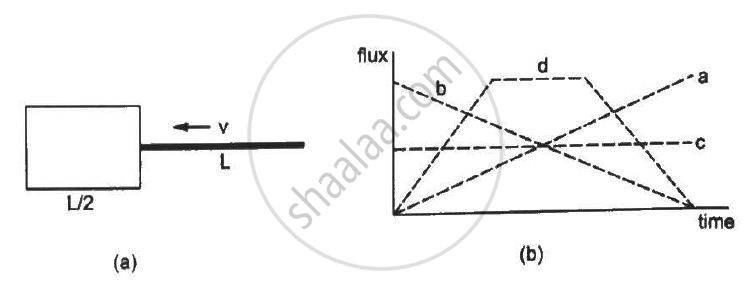• a

• b

• c

• d

MCQ | Q 7 | Page 140

A charge q is placed at the centre of the open end of a cylindrical vessel (see the figure). The flux of the electric field through the surface of the vessel is ____________ .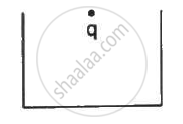•  zero

• q/εv

• q/2εv

• 2q/εv

MCQ [Pages 140 - 141]

### HC Verma solutions for Class 11, Class 12 Concepts of Physics Vol. 2 Chapter 8 Gauss’s Law MCQ [Pages 140 - 141]

MCQ | Q 1 | Page 140

Mark the correct options:

• Gauss's Law is valid only for symmetrical charge distributions.

• Gauss's Law is valid only for charges placed in vacuum.

•  The electric field calculated by Gauss's Law is the field due to the charge inside the Gaussian surface.

•  The flux of the electric field through a closed surface due to all the charges is equal to the flux due to the charges enclosed by the surface.

MCQ | Q 2 | Page 140

A positive point charge Q is brought near an isolated metal cube.

•  The cube becomes negatively charged.

• The cube becomes positively charged.

•  The interior becomes positively charged and the surface becomes negatively charged.

• The interior remains charge free and the surface gets non-uniform charge distribution.

MCQ | Q 3 | Page 140

A large non-conducting sheet M is given a uniform charge density. Two uncharged small metal rods A and B are placed near the sheet as shown in the following  figure.

(a) M attracts A.
(b) M attracts B.
(c) A attracts B.
(d) B attracts A.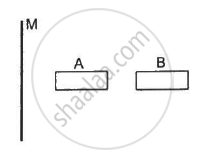MCQ | Q 4 | Page 140

If the flux of the electric field through a closed surface is zero,

(a) the electric field must be zero everywhere on the surface
(b) the electric field may be zero everywhere on the surface
(c) the charge inside the surface must be zero
(d) the charge in the vicinity of the surface must be zero

MCQ | Q 5 | Page 140

An electric dipole is placed at the centre of a sphere. Mark the correct options.
(a) The flux of the electric field through the sphere is zero.
(b) The electric field is zero at every point of the sphere.
(c) The electric field is not zero anywhere on the sphere.
(d) The electric field is zero on a circle on the sphere.

MCQ | Q 6 | Page 140

In the following figure shows a charge q placed at the centre of a hemisphere. A second charge Q is placed at one of the positions A, B, C and D. In which position(s) of this second charge, the flux of the electric field through the hemisphere remains unchanged?
(a) A
(b) B
(c) C
(d) D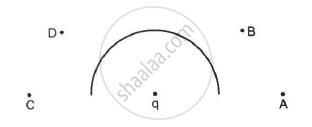MCQ | Q 7 | Page 140

A closed surface S is constructed around a conducting wire connected to a battery and a switch in the following figure. As the switch is closed, the free electrons in the wire start moving along the wire. In any time interval, the number of electrons entering the closed surface S is equal to the number of electrons leaving it. On closing the switch, the flux of the electric field through the closed surface
(a) is increased
(b) is decreased
(c) remains unchanged
(d) remains zero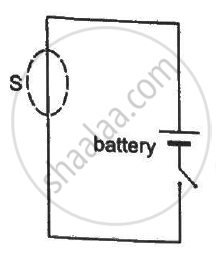MCQ | Q 8 | Page 141

The following figure shows a closed surface that intersects a conducting sphere. If a positive charge is placed at point P, the flux of the electric field through the closed surface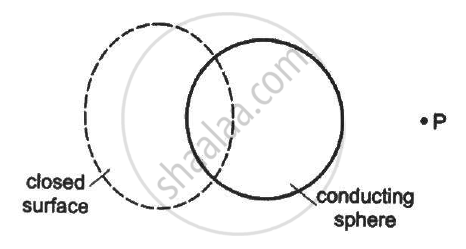•  will remain zero

• will become positive

•  will become negative

• will become undefined

[Pages 141 - 142]

### HC Verma solutions for Class 11, Class 12 Concepts of Physics Vol. 2 Chapter 8 Gauss’s Law [Pages 141 - 142]

Q 1 | Page 141

The electric field in a region is given by

vec"E"= 3/5"E"_0 vec"i" + 4/5 "E"_0 vec "i"  "with" " E"_0 = 2.0 xx 10^3 "N""C"^-1.

Find the flux of this field through a rectangular surface of area 0⋅2 m2 parallel to the y-z plane.

Q 2 | Page 141

A charge Q is uniformly distributed over a rod of length l. Consider a hypothetical cube of edge l with the centre of the cube at one end of the rod. Find the minimum possible flux of the electric field through the entire surface of the cube.

Q 3 | Page 141

Show that there can be no net charge in a region in which the electric field is uniform at all points.

Q 4 | Page 141

The electric field in a region is given by vec"E" = ("E"_0 "x")/"l"  vec"i".

Find the charge contained inside the cubical volume bound by the surfaces

x =0, x =a, y=0, y=a, z=0 and z=a. Take

"E"_0 = 5 xx 10^3 "N""C"^-1 , "l" =2 "cm" " and" " a" = 1 "cm"

Q 5 | Page 141

A charge Q is placed at the centre of a cube. Find the flux of the electric field through the six surfaces of the cube.

Q 6 | Page 141

A charge Q is placed at a distance a/2 above the centre of a horizontal, square surface of edge a as shown in the following figure . Find the flux of the electric field through the square surface.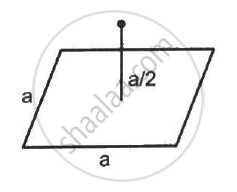Q 7 | Page 141

Find the flux of the electric field through a spherical surface of radius R due to a charge of 10−7 C at the centre and another equal charge at a point 2R away from the centre in the following figure.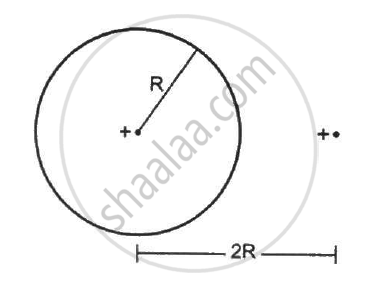Q 8 | Page 141

A charge Q is placed at the centre of an imaginary hemispherical surface. Using symmetry arguments and Gauss's Law, find the flux of the electric field due to this charge through the surface of the hemisphere in the following figure.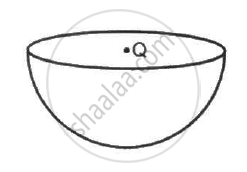Q 9 | Page 141

A spherical volume contains a uniformly distributed charge of density  2.0 × 10 -4 Cm-3 Find the electric field at a point inside the volume at a distance 4⋅0 cm from the centre.

Q 10 | Page 141

The radius of a gold nucleus (Z = 79) is about 7.0 × 10-10 m. Assume that the positive charge is distributed uniformly throughout the nuclear volume. Find the strength of the electric field at (a) the surface of the nucleus and (b) at the middle point of a radius. Remembering that gold is a conductor, is it justified to assume that the positive charge is uniformly distributed over the entire volume of the nucleus and does not come to the outer surface?

Q 11 | Page 141

A charge Q is distributed uniformly within the material of a hollow sphere of inner and outer radii r1 and r2 (see the figure). Find the electric field at a point P at a distance x away from the centre for r1 < x < r. Draw a rough graph showing the electric field as a function of x for 0 < x < 2r2 (see the figure).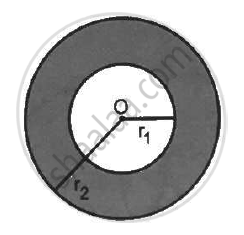Q 12 | Page 142

A charge Q is placed at the centre of an uncharged, hollow metallic sphere of radius a. (a) Find the surface. (b) If a charge q is put on the sphere, what would be the surface charge densities on the inner and outer surfaces? (c) Find the electric field inside the sphere at a distance x from the centre in the situations (a) and (b).

Q 13 | Page 142

Consider the following very rough model of a beryllium atom. The nucleus has four protons and four neutrons confined to a small volume of radius 10−15 m. The two 1 selectrons make a spherical charge cloud at an average distance of 1⋅3 ×10−11 m from the nucleus, whereas the two 2 s electrons make another spherical cloud at an average distance of 5⋅2 × 10−11 m from the nucleus. Find three electric fields at (a) a point just inside the 1 s cloud and (b) a point just inside the 2 s cloud.

Q 14 | Page 142

Find the magnitude of the electric field at a point 4 cm away from a line charge of density 2 × 10-6 Cm-1.

Q 15 | Page 142

A long cylindrical wire carries a positive charge of linear density  2.0 × 10-8 C      m -1 An electron revolves around it in a circular path under the influence of the attractive electrostatic force. Find the kinetic energy of the electron. Note that it is independent of the radius.

Q 16 | Page 142

A long cylindrical volume contains a uniformly distributed charge of density ρ. Find the electric field at a point P inside the cylindrical volume at a distance x from its axis (see the figure).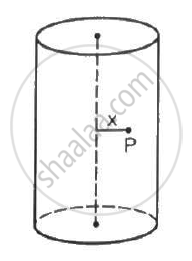Q 17 | Page 142

A non-conducting sheet of large surface area and thickness d contains a uniform charge distribution of density ρ. Find the electric field at a point P inside the plate, at a distance x from the central plane. Draw a qualitative graph of E against x for 0 < x < d.

Q 18 | Page 142

A charged particle with a charge of −2⋅0 × 10−6 C is placed close to a non-conducting plate with a surface charge density of 4.0 × 10-6Cm0-2. Find the force of attraction between the particle and the plate.

Q 19 | Page 142

One end of a 10 cm long silk thread is fixed to a large vertical surface of a charged non-conducting plate and the other end is fastened to a small ball of mass 10 g and a charge of 4.0× 10-6 C. In equilibrium, the thread makes an angle of 60° with the vertical. Find the surface charge density on the plate.

Q 20 | Page 142

One end of a 10 cm long silk thread is fixed to a large vertical surface of a charged non-conducting plate and the other end is fastened to a small ball of mass 10 g and a charge of 4.0× 10-6 C. In equilibrium, the thread makes an angle of 60° with the vertical (a) Find the tension in the string in equilibrium. (b) Suppose the ball is slightly pushed aside and released. Find the time period of the small oscillations.

Q 21 | Page 142

Two large conducting plates are placed parallel to each other with a separation of 2⋅00 cm between them. An electron starting from rest near one of the plates reaches the other plate in 2⋅00 microseconds. Find the surface charge density on the inner surfaces.

Q 22 | Page 142

Two large conducting plates are placed parallel to each other and they carry equal and opposite charges with surface density σ as shown in the figure. Find the electric field (a) at the left of the plates (b) in between the plates and (c) at the right of the plates.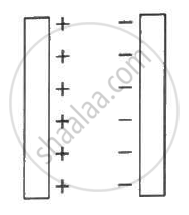Q 23 | Page 142

Two conducting plates X and Y, each with a large surface area A (on one side), are placed parallel to each other, as shown in the  following figure . Plate X is given a charge Q,whereas the other is kept neutral. Find (a) the surface charge density at the inner surface of plate X (b) the electric field at a point to the left of the plates (c) the electric field at a point in between the plates and (d) the electric field at a point to the right of the plates.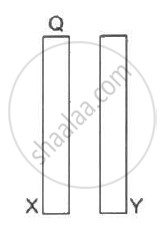Q 24 | Page 142

Three identical metal plates with large surface areas are kept parallel to each other as shown in the following figure. The leftmost plate is given a charge Q, the rightmost a charge −2Q and the middle one is kept neutral. Find the charge appearing on the outer surface of the rightmost plate.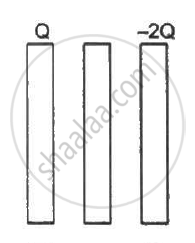## Chapter 8: Gauss’s Law

OthersMCQ## HC Verma solutions for Class 11, Class 12 Concepts of Physics Vol. 2 chapter 8 - Gauss’s Law

HC Verma solutions for Class 11, Class 12 Concepts of Physics Vol. 2 chapter 8 (Gauss’s Law) include all questions with solution and detail explanation. This will clear students doubts about any question and improve application skills while preparing for board exams. The detailed, step-by-step solutions will help you understand the concepts better and clear your confusions, if any. Shaalaa.com has the CBSE Class 11, Class 12 Concepts of Physics Vol. 2 solutions in a manner that help students grasp basic concepts better and faster.

Further, we at Shaalaa.com provide such solutions so that students can prepare for written exams. HC Verma textbook solutions can be a core help for self-study and acts as a perfect self-help guidance for students.

Concepts covered in Class 11, Class 12 Concepts of Physics Vol. 2 chapter 8 Gauss’s Law are Gauss’s Law, Physical Significance of Electric Field, Electric Field Due to a System of Charges, Charging by Induction, Electric Field Due to a Point Charge, Uniformly Charged Infinite Plane Sheet and Uniformly Charged Thin Spherical Shell (Field Inside and Outside), Applications of Gauss’s Law, Electric Flux, Dipole in a Uniform External Field, Electric Dipole, Electric Field Lines, Introduction of Electric Field, Continuous Charge Distribution, Superposition Principle of Forces, Forces Between Multiple Charges, Force Between Two Point Charges, Coulomb’s Law, Basic Properties of Electric Charge, Electric Charges.

Using HC Verma Class 12 solutions Gauss’s Law exercise by students are an easy way to prepare for the exams, as they involve solutions arranged chapter-wise also page wise. The questions involved in HC Verma Solutions are important questions that can be asked in the final exam. Maximum students of CBSE Class 12 prefer HC Verma Textbook Solutions to score more in exam.

Get the free view of chapter 8 Gauss’s Law Class 12 extra questions for Class 11, Class 12 Concepts of Physics Vol. 2 and can use Shaalaa.com to keep it handy for your exam preparation